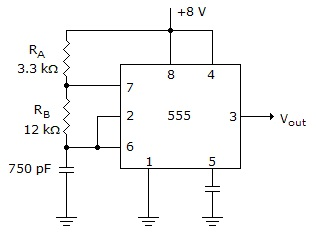# Digital Electronics - Multivibrators and 555 Timer - Discussion

### Discussion :: Multivibrators and 555 Timer - General Questions (Q.No.15)

15.

If a diode is connected across resistor RB (positive end up) in the given figure, what is the new duty cycle of the output waveform?[A]. 56% [B]. 44% [C]. 21.6% [D]. 17.4%

Explanation:

No answer description available for this question.

 Amita said: (May 6, 2011) What is duty cycle and how to solve this numerical? give full calculation.

 Manju said: (Sep 7, 2011) Tm=0.7*R1*C, Ts=0.7*R2*C duty cycle= R1/(R1+R2) or Tm/(Tm+Ts)

 A Banerjee said: (Sep 24, 2011) Ees ths is correct. If we want 50% duty cycle this is perfect ckt..only one 10k pot'll be connected betwn Ra & Rb., because for 50% duty cycle Ra must be equal zero. If we directly connected pin 7 to Vcc ckt may be damaged. (down transistor damaged/)

 Madhukar said: (May 25, 2013) When we connect a diode, the main purpose is to get the duty cycle < 0.5. Before connecting the diode the D = (RA+RB)/(RA+2RB). By the time we connect diode, the D becomes RA/ (RA+RB).

 Aimal said: (Dec 17, 2014) If you want less then 50% duty cycle then Rb is bypassed by a diode. This left out 56% out of box. The duty cycle can now be calculated (in this configuration) as: D.C = [Ra/Ra+Rb]*100.

 Kiran Borude said: (Sep 26, 2016) 3.3/(3.3 + 12) * 100 = 21.56%.

 Kiran Borude said: (Sep 26, 2016) Duty cycle = Ra + Rb/Ra + 2 * Rb. (3.3 + 12) * 10^6/(3.3 + 2 * 12) * 10^6. (3.3 + 12)/(3.3 + 2 * 12). 15.3/27.3 = .56 = 56%.